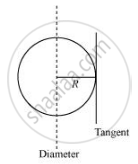# Find the Moment of Inertia of a Sphere About a Tangent to the Sphere, Given the Moment of Inertia of the Sphere About Any of Its Diameters to Be 2mr2/5, Where M is the Mass of the Sphere and R is the Radius of the Sphere. - Physics

Find the moment of inertia of a sphere about a tangent to the sphere, given the moment of inertia of the sphere about any of its diameters to be 2MR2/5, where is the mass of the sphere and is the radius of the sphere.

#### Solution 1

7/5 MR^2

The moment of inertia (M.I.) of a sphere about its diameter = 2/5 MR^2ML = 2/5MR^2

According to the theorem of parallel axes, the moment of inertia of a body about any axis is equal to the sum of the moment of inertia of the body about a parallel axis passing through its centre of mass and the product of its mass and the square of the distance between the two parallel axes.

The M.I. about a tangent of the sphere = 2/5 MR^2 + MR^2 = 7/5 MR^2

#### Solution 2

Moment of inertia of sphere about any diameter = 2/5 MR2

Applying theorem of parallel axes, Moment of inertia of sphere about a tangent to the sphere = 2/5MR2 +M(R)2 =7/5 MR2

Concept: Moment of Inertia
Is there an error in this question or solution?

#### APPEARS IN

NCERT Class 11 Physics
Chapter 7 System of Particles and Rotational Motion
Q 10.1 | Page 178